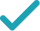Type a math problemSolve for mSteps Using the Quadratic Formula
Steps for Completing the Square
Variable cannot be equal to since division by zero is not defined. Multiply both sides of the equation by , the least common multiple of .
Multiply and to get .
Use the distributive property to multiply by .
Subtract from both sides.
Subtract from both sides.
Combine and to get .
All equations of the form can be solved using the quadratic formula: . The quadratic formula gives two solutions, one when is addition and one when it is subtraction.
This equation is in standard form: . Substitute for , for , and for in the quadratic formula, .
Square .
Multiply times .
Multiply times .
Take the square root of .
The opposite of is .
Multiply times .
Now solve the equation when is plus. Add to .
Divide by .
Now solve the equation when is minus. Subtract from .
Divide by .
The equation is now solved.
m=3mm+3\left(m-1\right)
Variable m cannot be equal to 0 since division by zero is not defined. Multiply both sides of the equation by 3m, the least common multiple of 3,m.
m=3m^{2}+3\left(m-1\right)
Multiply m and m to get m^{2}.
m=3m^{2}+3m-3
Use the distributive property to multiply 3 by m-1.
m-3m^{2}=3m-3
Subtract 3m^{2} from both sides.
m-3m^{2}-3m=-3
Subtract 3m from both sides.
-2m-3m^{2}=-3
Combine m and -3m to get -2m.
-2m-3m^{2}+3=0
-3m^{2}-2m+3=0
All equations of the form ax^{2}+bx+c=0 can be solved using the quadratic formula: \frac{-b±\sqrt{b^{2}-4ac}}{2a}. The quadratic formula gives two solutions, one when ± is addition and one when it is subtraction.
m=\frac{-\left(-2\right)±\sqrt{\left(-2\right)^{2}-4\left(-3\right)\times 3}}{2\left(-3\right)}
This equation is in standard form: ax^{2}+bx+c=0. Substitute -3 for a, -2 for b, and 3 for c in the quadratic formula, \frac{-b±\sqrt{b^{2}-4ac}}{2a}.
m=\frac{-\left(-2\right)±\sqrt{4-4\left(-3\right)\times 3}}{2\left(-3\right)}
Square -2.
m=\frac{-\left(-2\right)±\sqrt{4+12\times 3}}{2\left(-3\right)}
Multiply -4 times -3.
m=\frac{-\left(-2\right)±\sqrt{4+36}}{2\left(-3\right)}
Multiply 12 times 3.
m=\frac{-\left(-2\right)±\sqrt{40}}{2\left(-3\right)}
m=\frac{-\left(-2\right)±2\sqrt{10}}{2\left(-3\right)}
Take the square root of 40.
m=\frac{2±2\sqrt{10}}{2\left(-3\right)}
The opposite of -2 is 2.
m=\frac{2±2\sqrt{10}}{-6}
Multiply 2 times -3.
m=\frac{2\sqrt{10}+2}{-6}
Now solve the equation m=\frac{2±2\sqrt{10}}{-6} when ± is plus. Add 2 to 2\sqrt{10}\approx 6.32455532.
m=\frac{-\sqrt{10}-1}{3}
Divide 2+2\sqrt{10}\approx 8.32455532 by -6.
m=\frac{2-2\sqrt{10}}{-6}
Now solve the equation m=\frac{2±2\sqrt{10}}{-6} when ± is minus. Subtract 2\sqrt{10}\approx 6.32455532 from 2.
m=\frac{\sqrt{10}-1}{3}
Divide 2-2\sqrt{10}\approx -4.32455532 by -6.
m=\frac{-\sqrt{10}-1}{3} m=\frac{\sqrt{10}-1}{3}
The equation is now solved.
m=3mm+3\left(m-1\right)
Variable m cannot be equal to 0 since division by zero is not defined. Multiply both sides of the equation by 3m, the least common multiple of 3,m.
m=3m^{2}+3\left(m-1\right)
Multiply m and m to get m^{2}.
m=3m^{2}+3m-3
Use the distributive property to multiply 3 by m-1.
m-3m^{2}=3m-3
Subtract 3m^{2} from both sides.
m-3m^{2}-3m=-3
Subtract 3m from both sides.
-2m-3m^{2}=-3
Combine m and -3m to get -2m.
-3m^{2}-2m=-3
Quadratic equations such as this one can be solved by completing the square. In order to complete the square, the equation must first be in the form x^{2}+bx=c.
\frac{-3m^{2}-2m}{-3}=\frac{-3}{-3}
Divide both sides by -3.
m^{2}+\frac{-2}{-3}m=\frac{-3}{-3}
Dividing by -3 undoes the multiplication by -3.
m^{2}+\frac{2}{3}m=\frac{-3}{-3}
Divide -2 by -3.
m^{2}+\frac{2}{3}m=1
Divide -3 by -3.
m^{2}+\frac{2}{3}m+\left(\frac{1}{3}\right)^{2}=1+\left(\frac{1}{3}\right)^{2}
Divide \frac{2}{3}\approx 0.666666667, the coefficient of the x term, by 2 to get \frac{1}{3}\approx 0.333333333. Then add the square of \frac{1}{3}\approx 0.333333333 to both sides of the equation. This step makes the left hand side of the equation a perfect square.
m^{2}+\frac{2}{3}m+\frac{1}{9}=1+\frac{1}{9}
Square \frac{1}{3}\approx 0.333333333 by squaring both the numerator and the denominator of the fraction.
m^{2}+\frac{2}{3}m+\frac{1}{9}=\frac{10}{9}